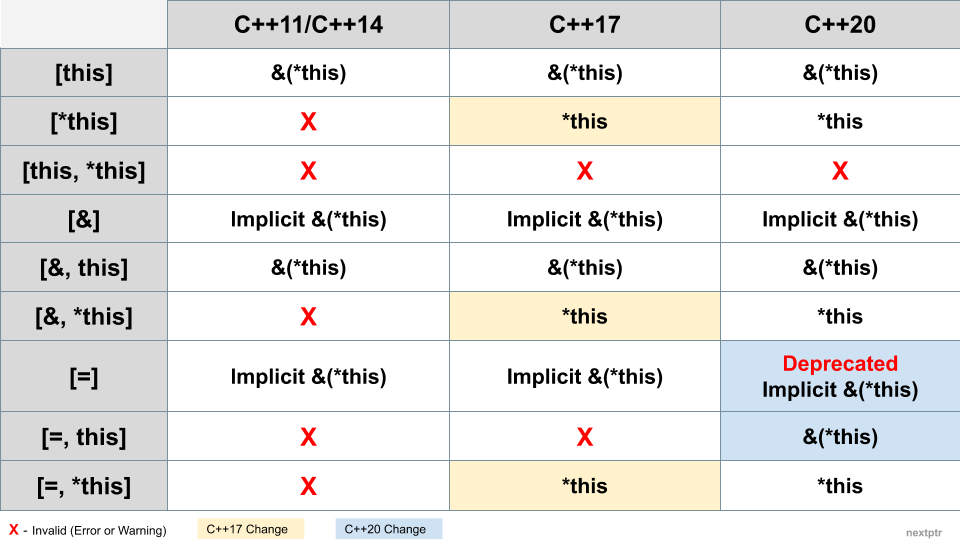# Capture *this in lambda expression: Timeline of change## 1. In Short

A lambda defined inside a non-static member function can directly access the members of the current object (or its copy) via an appropriate capture clause. But how the current object can be captured has gone through some changes since C++11.

Here, *this means the current object, and therefore &(*this) denotes a reference to the current object. Also, if a capture clause implicitly or explicitly captures this, conceptually, it captures &(*this).

Following is a list of captures relevant to capturing *this since C++11. Note that the below captures can include other variables also from the lambda's context (e.g., `[this]` could be `[this, i, j]`), as permitted. We are keeping it simple here for the sake of brevity.

• `[this]` — Captures *this by reference or &(*this).
• `[*this]`— Captures *this by value. By-value capture of *this is introduced in C++17.
• `[this, *this]` — Invalid. Cannot have this more than once.
• `[&]` — The reference-capture-default can implicitly capture this or &(*this).
• `[&, this]` — Same as `[&]`, therefore redundant.
• `[&, *this]` — Valid since C++17, but unlikely to be used.
• `[=]` — The value-capture-default can implicitly capture this or &(*this). However, the implicit capture of this through `[=]` is deprecated in C++20.
• `[=, this]` — Valid only since C++20. C++20 deprecates the implicit capture of this via `[=]` and allows the explicit capture of this in combination with `[=]`.
• `[=, *this]` — Valid since C++17.

The following table summarizes it all:The next few sections have details of the changes introduced in different standards for capturing *this.

## 2. C++11/C++14

Lambda expressions can capture *this by reference in C++11 by `[this]`. The two capture-defaults`[&]` and `[=]` — implicitly capture *this by reference. Examples:

``````struct Foo {
int m_x = 0;
void func() {
int x = 0;

//Implcit capture 'this'
[&]() { /*access m_x and x*/ }();

//Redundant 'this'
[&, this]() { /*access m_x and x*/ }();

//Implcit capture 'this'
[=]() { /*access m_x and x*/ }();

//Error
[=, this]() { }();
}
};
``````

C++14 makes no changes to how *this can be captured. There is no direct way in C++11/C++14 to capture *this by value. The only way to capture the current object by value is through the workaround of creating a copy of it explicitly. That workaround is cleaner in C++14 because of the init-capture:

``````struct Baz {
int m_x = 0;
void func() {
[copy=*this]() {
std::cout << copy.m_x;
}();

[copy=*this]() mutable {
copy.m_x++;
}();
}
};
``````

## 3. C++17

C++17 introduces the by-value capture of *this. The proposal document P0018R3 argues that by-value capture of *this is significantly important for concurrent applications that need to do asynchronous dispatch of closures.

Consider the following lambda that captures this and executes asynchronously in a different thread. The this pointer might be invalidated by the time lambda runs:

``````struct Processor {
//Some state data..

std::future<void> process(/*args*/) {
//Pre-process...
//Do the data processing asynchronously
return
std::async(std::launch::async,
[=](/*data*/){
/*
'this' might be invalidated here
*/

//process data
});
}
};

auto caller() {
Processor p;
return p.process(/*args*/);
}
``````

By-value capture of *this can solve the above problem, but in the absence of that, workarounds are necessary. The C++14 workaround to capture an explicit copy of *this is error-prone when combined with `[=]`. Because `[=, copy=*this]` implicitly captures this, forgetting to prefix a class member with 'copy.' could become an evasive defect. As shown below:

``````struct Donut {
int x = 0, y = 0;
void func() {
[=, copy=*this]() {
//y is accidently &(*this).y below
std::cout << copy.x << " " << y;
}();
}
};
``````

Since C++17, it is very intuitive to capture *this by value, as follows:

``````struct Some {
int x = 0, y = 0;
void func() {
//...
[=, *this]() {
std::cout << x << " " << y;
//...
}();

[=, *this]() mutable {
x *= y;
//...
}();
}
};
``````

## 4. C++20

In C++17, there is no ambiguity when it comes to the reference-capture-default `[&]`. The `[&]` captures this implicitly, `[&, this]` is redundant, and `[&, *this]` is allowed.

However, the value-capture-default `[=]` is quite inconsistent. The `[=]` captures this implicitly, `[=, *this]` is not redundant, and `[=, this]` is not allowed. What one would expect is that `[=]` captures *this by-value implicitly, `[=, *this]` is redundant, and `[=, this]` is allowed.

To clear the inconsistency seems like the move is towards getting rid of the implicit capture altogether. Therefore, C++20 deprecates the implicit capture of this via `[=]` (P0806R2) and allows `[=, this]` (P0409R2). And it is possible that the other remaining implicit capture — of this via `[&]` — might be deprecated in the future.

In C++20, accessing class members in a lambda with `[=]` capture leads to a warning:

``````struct Bagel {
int x = 0;
void func() {
//...
//OK until C++20. Warning in C++20.
[=]() { std::cout << x; }();

//Error/warning until C++20. OK in C++20.
[=, this]() { std::cout << x; }();
}
};
``````